Select Page

# Maths Vector Algebra CBSE 12 Science Answers for MCQ in English

Maths Vector Algebra CBSE 12 Science Answers for MCQ in English to enable students to get Answers in a narrative video format for the specific question.

Expert Teacher provides Maths Vector Algebra CBSE 12 Science Answers for MCQ through Video Answers in English language. This video solution will be useful for students to understand how to write an answer in exam in order to score more marks. This teacher uses a narrative style for a question from Vector Algebra not only to explain the proper method of answering question, but deriving right answer too.

Please find the question below and view the Answer in a narrative video format.

Question:

## Similar Questions from CBSE, 12th Science, Maths, Vector Algebra

Question 1 :  Write the value of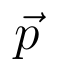for which the vectors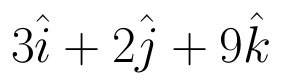and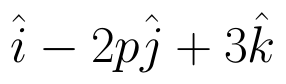are parallel vectors.  (View Answer Video)

Question 2 : Find a unit vector parallel to the sum of vectors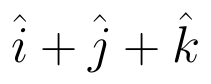and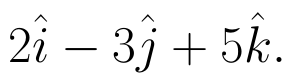(View Answer Video)

Question 3 : Find the scalar components of the vector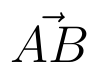with initial point A (2, 1) and terminal point B (-5, 7).  (View Answer Video)

Question 4 :  P and Q are two points with position vectors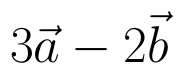and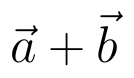respectively. Write the position vector of a point R which divides the line segment PQ externally in the ratio 2:1.  (View Answer Video)

Question 5 :  Find the scalar quantities from the following:
(i) 10 kg          (ii) 2 m north-west          (iii)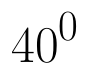(iv) 40 W         (v)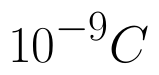(vi)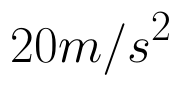(View Answer Video)

### Continuity and Differentiability

Question 1 : If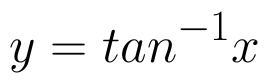, find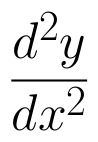in terms of y alone. (View Answer Video)

Question 2 : If x and y are connected parametrically by the equation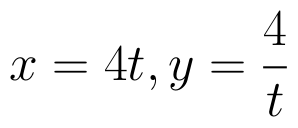, without eliminating the parameter, find. (View Answer Video)

Question 3 : Differentiate the function w.r.t.x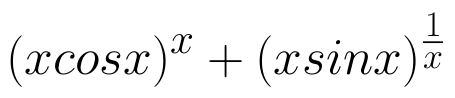. (View Answer Video)

Question 4 : Find the value of k, if the area of the triangle is 4 sq unit and vertices are (-2, 0), (0, 4), (0, k). (View Answer Video)

Question 5 : Differentiate the function w.r.t.x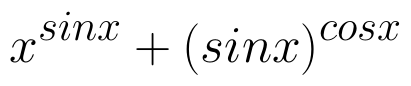. (View Answer Video)

### Inverse Trigonometric Functions

Question 1 : Write the value of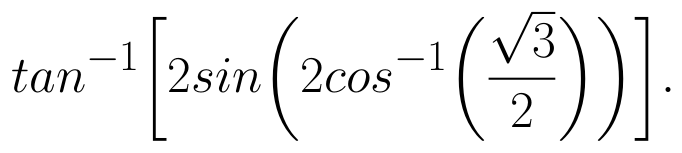(View Answer Video)

Question 2 : Write the principal value of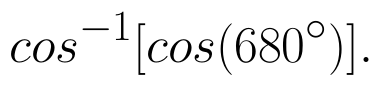(View Answer Video)

Question 3 :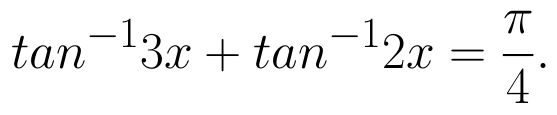What value do you observe in real life scenario ? (View Answer Video)

Question 4 : Evaluate :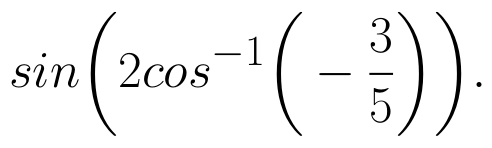(View Answer Video)

Question 5 : Write the value of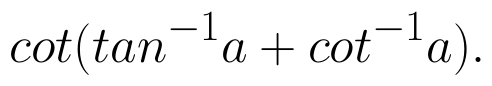(View Answer Video)

### Application of Derivatives

Question 1 : The radius of an air bubble is increasing at the rate of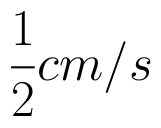. At what rate is the volume of the bubble increasing when its radius is 1 cm? (View Answer Video)

Question 2 : The normal at the point (1, 1) on the curve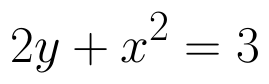is: (View Answer Video)

Question 3 : The line y = mx + 1 is a tangent to the curve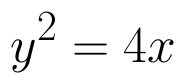if the value of m is: (View Answer Video)

Question 4 : The function f is a differentiable function and satisfies the functional equation f(x) + f(y) = f(x + y) – xy – 1for every pair x, y of real numbers. If f(1) = 1, then the number of integers n ≠ 1 for which f(n) = n is_______________. (View Answer Video)

Question 5 : A cylindrical tank of radius 10 m is being filled with wheat at the rate of the 314 cubic meters per hour. Then the depth of the wheat is increasing at the rate of ___________. (View Answer Video)